# Book: A Course in Enumeration. Author: Martin Aigner Chapter 1 Page:60 2.4 Let F(2)-「20 anz" with a0-0. Show that...

Book: A Course in Enumeration. Author: Martin Aigner

Chapter 1 Page:60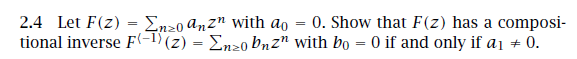2.4 Let F(2)-「20 anz" with a0-0. Show that F(z) has a composi- tional inverse F(-1) (2)-Ση20Pnz" with bo 0 if and only if ai + 0. , 71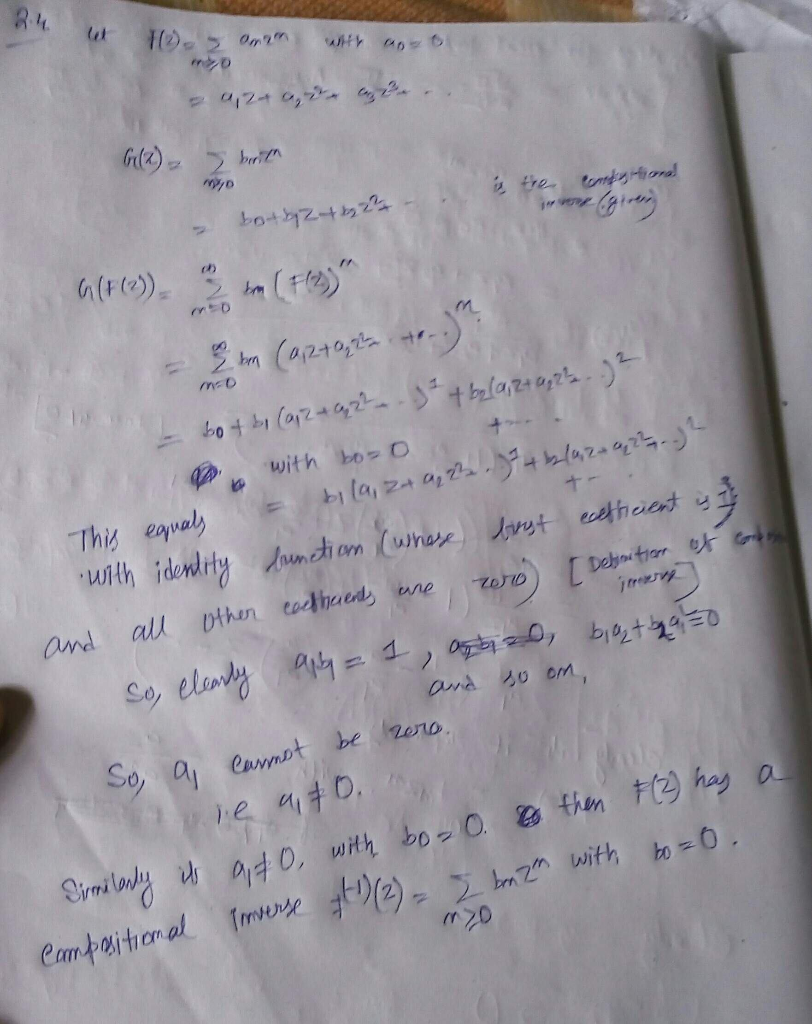##### Add Answer of: Book: A Course in Enumeration. Author: Martin Aigner Chapter 1 Page:60 2.4 Let F(2)-「20 anz" with a0-0. Show that...
Similar Homework Help Questions
• ### Book: A Course in Enumeration. Author: Martin Aigner Chapter 1 Page:42 n- k n- k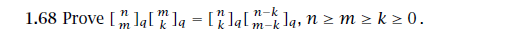Book: A Course in Enumeration. Author: Martin Aigner Chapter 1 Page:42 n- k n- k

• ### Book: A Course in Enumeration. Author: Martin Aigner Chapter 1 Page:60 If A(z) = n≥0 , then an is the n-th coefficient...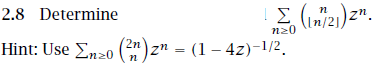Book: A Course in Enumeration. Author: Martin Aigner Chapter 1 Page:60 If A(z) = n≥0 , then an is the n-th coefficient of A(z), for which we also write = []A(z). Sometimes it is useful to consider coefficients with negative index, with the understanding that ak = 0 for k < 0. The coefficient a0 is called the constant coefficient, and we also write a0 = A(0). The set of all formal series over C shall be denoted by C[[z]]....

• ### Book: A Course in Enumeration. Author: Martin Aigner Chapter 1 Page:35 1.59 Determine the number of solutions of XI +··...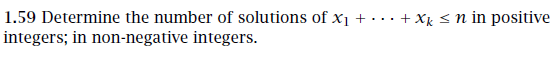Book: A Course in Enumeration. Author: Martin Aigner Chapter 1 Page:35 1.59 Determine the number of solutions of XI +···+ Xk integers; in non-negative integers. n in positive Show transcribed image text

• ### Book: A Course in Enumeration. Author: Martin Aigner Chapter 2 Page:76 2.41 Derive the 2-term recurrence...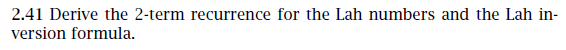Book: A Course in Enumeration. Author: Martin Aigner Chapter 2 Page:76 2.41 Derive the 2-term recurrence for the Lah numbers and the Lah in- version formula 2.41 Derive the 2-term recurrence for the Lah numbers and the Lah in- version formula

• ### Book: A Course in Enumeration. Author: Martin Aigner Chapter 1 Page:29 S(n) is the set of all permutations of {1, 2, ....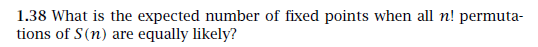Book: A Course in Enumeration. Author: Martin Aigner Chapter 1 Page:29 S(n) is the set of all permutations of {1, 2, . . . ,n}. 1.38 What is the expected number of fixed points when all n! permut:a tions of S(n) are equally likely? Show transcribed image text

• ### Book: A Course in Enumeration. Author: Martin Aigner Chapter 1 Page:35 1.60 Let e(n),o(n), and sc(n) denote, respective...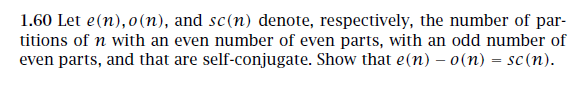Book: A Course in Enumeration. Author: Martin Aigner Chapter 1 Page:35 1.60 Let e(n),o(n), and sc(n) denote, respectively, the number of par- titions of n with an even number of even parts, with an odd number of even parts, and that are self-conjugate. Show that e(n) - o(n) - sc(n). Show transcribed image text

• ### Book: A Course in Enumeration. Author: Martin Aigner Chapter 1 Page:42 1.72 Prove the q-Vandermonde identity 72 (r-k) (...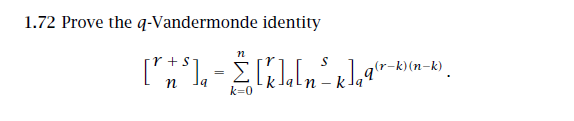Book: A Course in Enumeration. Author: Martin Aigner Chapter 1 Page:42 1.72 Prove the q-Vandermonde identity 72 (r-k) (n-k) k=0 1.72 Prove the q-Vandermonde identity 72 (r-k) (n-k) k=0

• ### Book: A Course in Enumeration. Author: Martin Aigner Chapter 1 Page:29 1.41 Letl2. Show that the number of permutations...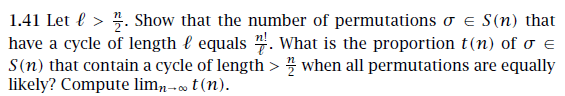Book: A Course in Enumeration. Author: Martin Aigner Chapter 1 Page:29 1.41 Letl2. Show that the number of permutations a e S(n) that have a cycle of length l equals . What is the proportion t(n) of o e S(n) that contain a cycle of length > when all permutations are equally likely? Compute lim,- t(n) 1.41 Letl2. Show that the number of permutations a e S(n) that have a cycle of length l equals . What is the proportion...

• ### Book: A Course in Enumeration. Author: Martin Aigner Chapter 1 Page:29 1.37 Use the polynomial method to show that sn lkti -o )sni Can you find a combinatorial proof? 1.37 Use the polynomial met...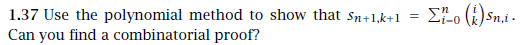Book: A Course in Enumeration. Author: Martin Aigner Chapter 1 Page:29 1.37 Use the polynomial method to show that sn lkti -o )sni Can you find a combinatorial proof? 1.37 Use the polynomial method to show that sn lkti -o )sni Can you find a combinatorial proof?

• ### Book: A Course in Enumeration. Author: Martin Aigner Chapter 1 Page:29 According to this chapter, I think S n,k is the Stirling number and maybe the first kind. 1.37 Use the polynomial method to show...Book: A Course in Enumeration. Author: Martin Aigner Chapter 1 Page:29 According to this chapter, I think S n,k is the Stirling number and maybe the first kind. 1.37 Use the polynomial method to show that sn lkti -o )sni Can you find a combinatorial proof? 1.37 Use the polynomial method to show that sn lkti -o )sni Can you find a combinatorial proof?

Need Online Homework Help?